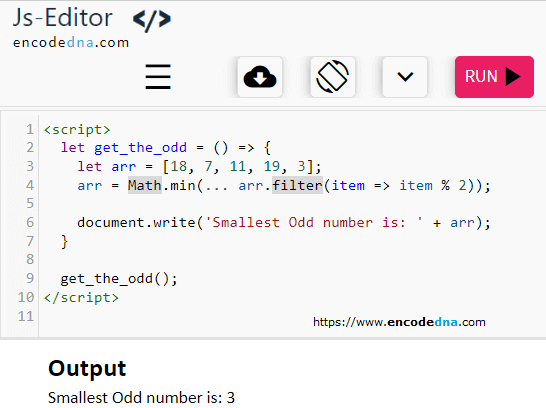﻿ JavaScript - How to find the Smallest Odd number in an Array

# JavaScript - How to find the Smallest Odd number in an Array

It’s super easy. You can use the filter and Math function in JavaScript to find the smallest Odd number in an array.

Heres' a one-liner JS code.

```<script>
let get_the_odd = () => {
let arr = [18, 7, 11, 19, 3];
arr = Math.min(... arr.filter(item => item % 2));       //  one-liner JS code.
document.write(arr);
}

get_the_odd();
</script>```Now, let me break the code for you. First, it will filter out all the odd numbers in the array. Next, it finds the minimum value or the smallest odd number in the array.

```<script>
let arr = [18, 7, 11, 19, 3];
arr = arr.filter(item => item % 2);       // filter out all odd numbers.
document.write(Math.min(... arr));        // using spread operator with Math.min() method.
</script>```

Similarly, you can easily filter out only numbers in an Array using JavaScript.

Another filter method example... How to filter only 4 letter words from an array in JavaScript.
I created a tool called Random Word Generator using the methods shown in this article.

That's it. Happy coding. 🙂